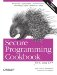# Getting the Minimum and Maximum Values for a Numeric Type

Problem

You need to know the largest or smallest representable value for your platform for a numeric type, such as an int or double.

Solution

Use the numeric_limits class template in the header to get, among other things, the largest and smallest possible values for a numeric type (see Example 3-9).

Example 3-9. Getting numeric limits

```#include
#include

using namespace std;

template
void showMinMax( ) {
cout << "min: " << numeric_limits::min( ) << endl;
cout << "max: " << numeric_limits::max( ) << endl;
cout << endl;
}

int main( ) {

cout << "short:" << endl;
showMinMax( );
cout << "int:" << endl;
showMinMax( );
cout << "long:" << endl;
showMinMax( );
cout << "float:" << endl;
showMinMax( );
cout << "double:" << endl;
showMinMax( );
cout << "long double:" << endl;
showMinMax( );
cout << "unsigned short:" << endl;
showMinMax( );
cout << "unsigned int:" << endl;
showMinMax( );
cout << "unsigned long:" << endl;
showMinMax( );
}```

Here's what I get on Windows XP using Visual C++ 7.1:

```short:
min: -32768
max: 32767

int:
min: -2147483648
max: 2147483647

long:
min: -2147483648
max: 2147483647

float:
min: 1.17549e-038
max: 3.40282e+038

double:
min: 2.22507e-308
max: 1.79769e+308

long double:
min: 2.22507e-308
max: 1.79769e+308

unsigned short:
min: 0
max: 65535

unsigned int:
min: 0
max: 4294967295

unsigned long:
min: 0
max: 4294967295```

Discussion

Example 3-9 shows a simple example for getting the minimum and maximum values for native numeric types. The numeric_limits class template has a specialization for all of the built-in types, including both numeric and nonnumeric. The standard mandates that all of the types I use in Example 3-9 have a specialization of numeric_limits, as well as these:

```bool
char
signed char
unsigned char
wchar_t```

min and max are static member functions in numeric_limits that return the highest and lowest values for the type parameter you pass in.Secure Programming Cookbook for C and C++: Recipes for Cryptography, Authentication, Input Validation & More
ISBN: 0596003943
EAN: 2147483647
Year: 2006
Pages: 241